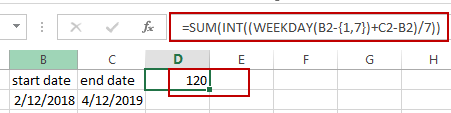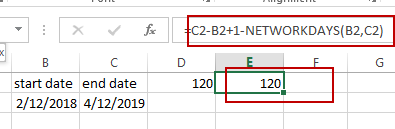# Count the Number of Weekends between Two Date

This post will guide you how to count the number of weekends between two dates in Excel. How do I get the number of weekends between 2 dates with a formula in Excel. How to count weekend days between dates in Excel.

## Count the Number of Weekends between Two dates

If you want to calculate the number of weekends between two dates, you need to create a formula based on the SUM function, the INT function and the WEEKDAY function. For example, you have two dates in Cell B2:C2, and the start date is in Cell B2, and the end date is in Cell C2. You can use the following formula to achieve the result.

`=SUM(INT((WEEKDAY(B2-{1,7})+C2-B2)/7))`

Type this formula into a blank cell and then press Enter key in your keyboard.The number of Weekends between those two dates is calculated.

Or you can use another formula to achieve the same result. Type the following formula into a blank cell and press Enter key.

`=C2-B2+1-NETWORKDAYS(B2,C2)`### Related Functions

• Excel SUM function
The Excel SUM function will adds all numbers in a range of cells and returns the sum of these values. You can add individual values, cell references or ranges in excel.The syntax of the SUM function is as below:= SUM(number1,[number2],…)…
• Excel INT function
The Excel INT function returns the integer portion of a given number. And it will rounds a given number down to the nearest integer.The syntax of the INT function is as below:= INT (number)…
• Excel WEEKDAY function
The Excel WEEKDAY function returns a integer value representing the day fo the week for a given Excel date and the value is range from 1 to 7.The syntax of the WEEKDAY function is as below:=WEEKDAY (serial_number,[return_type])…